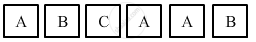Advertisement Remove all ads

# A Child Has a Die Whose 6 Faces Show the Letters Given Below: the Die is Thrown Once. What is the Probability of Getting (I) a and (Ii) B? - Mathematics

Short Note

A child has a die whose 6 faces show the letters given below:The die is thrown once. What is the probability of getting (i) A and (ii) B?

Advertisement Remove all ads

#### Solution

In a single throw of a die, the possible outcomes are:
A,B,C,A,A,B

Total number of possible outcomes = 6

(i) Let E1 be the event of getting A.

Number of favourable outcomes = 3
∴ P( getting A) =  P (E1) =  3/6=1/2

Concept: Concept Or Properties of Probability
Is there an error in this question or solution?
Advertisement Remove all ads

#### APPEARS IN

RS Aggarwal Secondary School Class 10 Maths
Chapter 15 Probability
Exercise 15A | Q 6 | Page 687
Advertisement Remove all ads

#### Video TutorialsVIEW ALL 

Advertisement Remove all ads
Share
Notifications

View all notifications

Forgot password?
Course